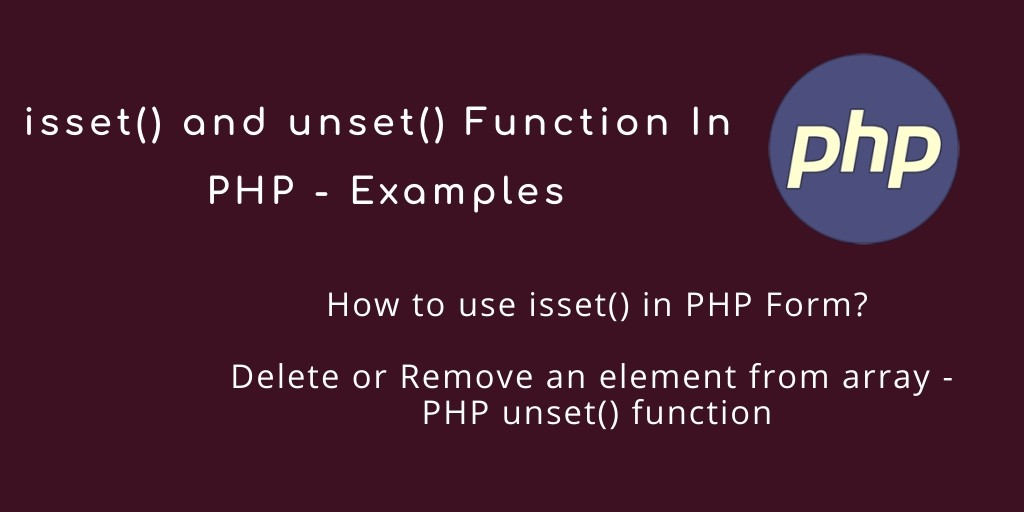Html5 27-Jul-2021

### isset() and unset() Function In PHP – Examples

PHP isset() and unset() Function. In this tutorial, we would love to share with what is PHP isset() and unset() function, how to use this isset and unset function of PHP.

Also, this tutorial teach you, how to use isset() in PHP Form and how to check array unset function in PHP.

You should also read this php post: PHP empty() – Check Variable is Empty in PHP

## isset and unset Function in PHP

Here you will learn how to use isset and unset function of PHP with its syntax and examples:

### PHP isset() Function

isset() function in PHP is used to check whether a variable is set or not. This means that it decides whether a variable is assigned a value and is not null.

#### Syntax – PHP isset() function

The basic Syntax of PHP isset() function is:

`isset(\$variable);`

Note:- If use isset() function in PHP, PHP isset() function returns true if a variable is set and if it is not set it will be false.

#### Example – PHP isset Function

Let’s take an example, Here we will simply declare a string variable and assigned it value. and will use the isset function of php for checking it’s set or not.

<?php

\$issetVal = "";

If (isset(\$issetVal)){
echo "The variable is set";
}
else {
echo "The variable is not set";
}

?>

##### Source code of isset function In PHP

<!DOCTYPE html>
<html>
<title>How to check a variable set or not in php | PHP Tutorial</title>
<body>

<h4>How to check a variable set or not in php</h4>

<?php

\$issetVal = "";

if(isset(\$issetVal)){
echo "Result:- The variable is set";
}
else {
echo "Result:- The variable is not set";
}

?>

</body>
</html>

#### Second Example Of PHP isset() function

Let’s take a second example of php isset() function. In this example, we will not assign it any value or variable. We have just declared that string variable and see the output:

<?php
\$issetVal;

if(isset(\$issetVal)){
echo "Result:- The variable is set";
}
else {
echo "Result:- The variable is not set";
}
?>

Source code of Second Example – PHP isset function

<!DOCTYPE html>
<html>
<title>How to check a variable set or not in php | PHP Tutorial</title>
<body>

<h4>How to check a variable set or not in php</h4>

<?php

\$issetVal;

if(isset(\$issetVal)){
echo "Result:- The variable is set";
}
else {
echo "Result:- The variable is not set";
}

?>

</body>
</html>

### PHP unset() Function

In PHP, unset() function is used to destroy a defined variable. That means this function unsets only local variable. If we want to unset the global variable inside the function then we have to use \$GLOBALS array to do so.

#### Syntax – PHP unset() function

The basic Syntax of PHP unset() function is:

`unset(\$variable_name);`

Note: When you use the PHP unset function, You may specify one or more variable to be destroyed by using the unset in a single call.

#### Example – PHP unset Function

Let’s take an example, Here we will simply declare a string variable and assigned it value. and will use the unset function of php for destroy it.

<?php

\$str = "This is simple string";

//Using unset function

unset (\$str);

//
If (isset(\$str)){
echo "The variable is set";
}
else {
echo "The variable is not set";
}

?>

##### Source Code of Unset Function In PHP

<!DOCTYPE html>
<html>
<title>Unset Variable In PHP using PHP unset() Function | PHP Tutorial</title>
<body>

<h4>Unset Variable In PHP using PHP unset() Function</h4>

<?php

\$str = "This is simple string";

//Using unset function

unset (\$str);

//
If (isset(\$str)){
echo "The variable is set";
}
else {
echo "The variable is not set";
}

?>

</body>
</html>

### Important Note of PHP unset() function

• If you want to destroy a global variable is inside a function, it will only be destroyed in that function. This will maintain the value on the outside.
• The unset function does not return any value.

## Faqs of PHP isset() and unset() function

#### 1. How to use isset() in PHP Form?

You can use the isset() function in PHP form like below:

<?php

if (isset(\$_POST['submit'])) {
echo "Hello, you have successfully check a variable using isset() function in PHP";

?>

##### Source Code of check isset() for PHP Form

<?php

if (isset(\$_POST['submit'])) {
echo "Hello, you have successfully check a variable using isset() function in PHP";

?>

<!DOCTYPE html>
<html>
<title>PHP Form with isset</title>

<body>
<form action="<?php echo htmlspecialchars(\$_SERVER["PHP_SELF"]); ?>" method="post">

Name : <input type = "text" name = "name"
placeholder = "Enter Name" />

<br><br>

Email : <input type = "text"
name = "email" placeholder = "Enter Email" />

<br><br>

<input type = "submit" name = "submit" value = "Submit">
</form>
</body>
</html>

#### 2. Delete or Remove an element from array – PHP unset() function

if you have array and want to remove or delete an element of array. So you can use the php unset() function for removing and deleting an array item.

<?php

// You have array like this
\$arr = array('A', 'C', 'X','G', 'E', 'E', 'K', 'S');

// Display the array element
print_r(\$arr);

// Use PHP unset() function delete or remove array elements

// elements
unset(\$arr);

// Display the array element
print_r(\$arr);

?>

Source Code of Delete or Remove an element from array – PHP unset() function

<!DOCTYPE html>
<html>
<title>Delete or Remove an element from array - PHP unset() function | PHP Tutorial</title>
<body>

<h4>Delete or Remove an element from array - PHP unset() function</h4>

<?php

// You have array like this
\$arr = array('A', 'C', 'X','G', 'E', 'E', 'K', 'S');

// Display the array element
print_r(\$arr);

// Use PHP unset() function delete or remove array elements
unset(\$arr);

echo "<br>";
// Display the array element
print_r(\$arr);

?>

</body>
</html>